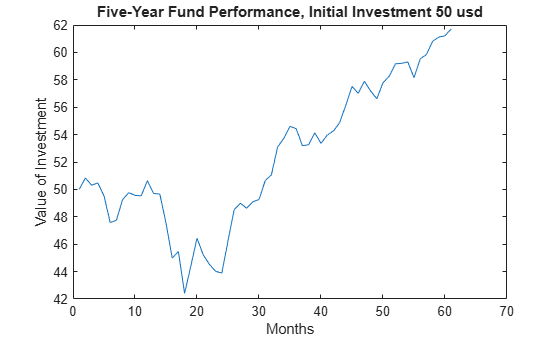# maxdrawdown

Compute maximum drawdown for one or more price series

## Syntax

``MaxDD = maxdrawdown(Data)``
``MaxDD = maxdrawdown(___,Format)``
``[MaxDD,MaxDDIndex] = maxdrawdown(___)``

## Description

example

````MaxDD = maxdrawdown(Data)` computes maximum drawdown for each series in an `N`-vector `MaxDD` and identifies start and end indexes of maximum drawdown periods for each series in a `2`-by-`N` matrix `MaxDDIndex`.```

example

````MaxDD = maxdrawdown(___,Format)` adds an optional argument for `Format`.```

example

````[MaxDD,MaxDDIndex] = maxdrawdown(___)` adds an optional output for `MaxDDIndex`.```

## Examples

collapse all

Calculate the maximum drawdown (`MaxDD`) using example data with a fund, market, and cash series:

```load FundMarketCash MaxDD = maxdrawdown(TestData)```
```MaxDD = 1×3 0.1658 0.3381 0 ```

The maximum drop in the given time period was 16.58% for the fund series, and 33.81% for the market series. There was no decline in the cash series, as expected, because the cash account never loses value.

`maxdrawdown` also returns the indices (`MaxDDIndex`) of the maximum drawdown intervals for each series in an optional output argument.

`[MaxDD, MaxDDIndex] = maxdrawdown(TestData)`
```MaxDD = 1×3 0.1658 0.3381 0 ```
```MaxDDIndex = 2×3 2 2 NaN 18 18 NaN ```

The first two series experience their maximum drawdowns from the second to the 18th month in the data. The indices for the third series are `NaN`s because it never has a drawdown.

The 16.58% value loss from month 2 to month 18 for the fund series is verified using the reported indices.

```Start = MaxDDIndex(1,:); End = MaxDDIndex(2,:); (TestData(Start(1),1) - TestData(End(1),1))/TestData(Start(1),1)```
```ans = 0.1658 ```

`ans = 0.1658`

Although the maximum drawdown is measured in terms of returns, `maxdrawdown` can measure the drawdown in terms of absolute drop in value, or in terms of log-returns. To contrast these alternatives more clearly, you can work with the fund series, assuming an initial investment of 50 dollars:

```Fund50 = 50*TestData(:,1); plot(Fund50); title('\bfFive-Year Fund Performance, Initial Investment 50 usd'); xlabel('Months'); ylabel('Value of Investment');```First, compute the standard maximum drawdown, which coincides with the results above because returns are independent of the initial amounts invested.

`MaxDD50Ret = maxdrawdown(Fund50)`
```MaxDD50Ret = 0.1658 ```

Next, compute the maximum drop in value, using the `'arithmetic'` argument.

`[MaxDD50Arith, Ind50Arith] = maxdrawdown(Fund50,'arithmetic')`
```MaxDD50Arith = 8.4285 ```
```Ind50Arith = 2×1 2 18 ```

The value of this investment was \$50.84 in month 2, but by month 18 the value was down to \$42.41, a drop of \$8.43. This is the largest loss in dollar value from a previous high in the given time period. In this case, the maximum drawdown period, from the 2nd to 18th month, is the same independently of whether drawdown is measured as return or as dollar value loss.

`[MaxDD50LogRet, Ind50LogRet] = maxdrawdown(Fund50,'geometric')`
```MaxDD50LogRet = 0.1813 ```
```Ind50LogRet = 2×1 2 18 ```

Note, the last measure is equivalent to finding the arithmetic maximum drawdown for the log of the series.

`MaxDD50LogRet2 = maxdrawdown(log(Fund50),'arithmetic')`
```MaxDD50LogRet2 = 0.1813 ```

## Input Arguments

collapse all

Total return price series, specified as a `T`-by-`N` matrix with `T` samples of `N` total return price series.

Data Types: `double`

Format of `Data`, specified as character vector with the following possible values:

• `'return'` (default) — Maximum drawdown in terms of maximum percentage drop from a peak.

• `'arithmetic'`

— Maximum drawdown of an arithmetic Brownian motion with drift (differences of data from peak to trough) using the equation

`$dX\left(t\right)=\mu dt+\sigma dW\left(t\right).$`

• `'geometric'`

— Maximum drawdown of a geometric Brownian motion with drift (differences of log of data from peak to trough) using the equation

`$dS\left(t\right)={\mu }_{0}S\left(t\right)dt+{\sigma }_{0}S\left(t\right)dW\left(t\right)$`

Data Types: `char`

## Output Arguments

collapse all

Maximum drawdown, returned as a `1`-by-`N` vector with maximum drawdown for each of `N` time series.

Note

• Drawdown is the percentage drop in total returns from the start to the end of a period. If the total equity time series is increasing over an entire period, drawdown is 0. Otherwise, it is a positive number. Maximum drawdown is an ex-ante proxy for downside risk that computes the largest drawdown over all intervals of time that can be formed within a specified interval of time.

• Maximum drawdown is sensitive to quantization error.

Start and end indexes for each maximum drawdown period for each total equity time series, returned as a `2`-by-`N` vector of start and end indexes. The first row of the vector contains the start indexes and the second row contains the end indexes of each maximum drawdown period.

 Christian S. Pederson and Ted Rudholm-Alfvin. "Selecting a Risk-Adjusted Shareholder Performance Measure." Journal of Asset Management. Vol. 4, No. 3, 2003, pp. 152–172.